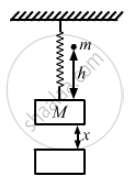Advertisement Remove all ads

# A Block of Mass 200 G is Suspended Through a Vertical Spring. the Spring is Stretched by 1.0 Cm When the Block is in Equilibrium. - Physics

Sum

A block of mass 200 g is suspended through a vertical spring. The spring is stretched by 1.0 cm when the block is in equilibrium. A particle of mass 120 g is dropped on the block from a height of 45 cm. The particle sticks to the block after the impact. Find the maximum extension of the spring. Take g = 10 m/s2.

Advertisement Remove all ads

#### Solution

It is given that:
Mass of block, M = 200 g = 0.20 kg
Mass of the particle, m = 120 gm = 0.12 kg
Height of the particle, h = 45 cm = 0.45 mAccording to question, as the block attains equilibrium, the spring is stretched by a distance, x = 1.00 cm = 0.01 m.

i.e.  M × g = K × x

⇒ 0.2 × g = K × x
⇒ 2 = K × 0.01
⇒ K = 200 N/m

The velocity with which the particle m strikes M is given by,

$u = \sqrt{2gh}$

$u = \sqrt{2 \times 10 \times 0 . 45}$

$= \sqrt{9} = 3 \text{ m/s}$
After the collision, let the velocity of the particle and the block be V.
According to law of conservation of momentum, we can write:
mu = (m + M)V
Solving for V , we get:
$V = \frac{0 . 12 \times 3}{0 . 32} = \frac{9}{8}\text{ m/s }$
Let the spring be stretched through an extra deflection of δ.
On applying the law of conservation of energy, we can write:
Initial energy of the system before collision = Final energy of the system
$\Rightarrow \frac{1}{2}m u^2 + \frac{1}{2}K x^2 = \frac{1}{2}\left( m + M \right) V^2 + \frac{1}{2}K \left( x + \delta \right)^2$
Substituting appropriate values in the above equation, we get:
$\left( \frac{1}{2} \right) \times 0 . 12 \times 9 + \left( \frac{1}{2} \right) \times 200 \times (0 . 01 )^2 = \left( \frac{1}{2} \right)0 . 32 \times \left( \frac{81}{64} \right) + \left( \frac{1}{2} \right) \times 200 \times (\delta + 0 . 1 )^2$
On solving the above equation, we get:
δ = 0.061
m = 6.1 cm

Concept: Momentum Conservation and Centre of Mass Motion
Is there an error in this question or solution?
Advertisement Remove all ads

#### APPEARS IN

HC Verma Class 11, 12 Concepts of Physics 1
Chapter 9 Centre of Mass, Linear Momentum, Collision
Q 46 | Page 162
Advertisement Remove all ads
Advertisement Remove all ads
Share
Notifications

View all notifications

Forgot password?